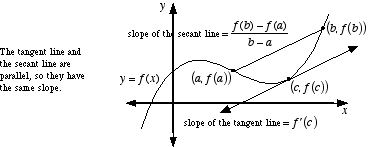index: click on a letter A B C D E F G H I J K L M N O P Q R S T U V W X Y Z A to Z index index: subject areas numbers & symbols sets, logic, proofs geometry algebra trigonometry advanced algebra & pre-calculus calculus advanced topics probability & statistics real world applications multimedia entrieswww.mathwords.com about mathwords website feedback

 Mean Value Theorem A major theorem of calculus that relates values of a function to a value of its derivative. Essentially the theorem states that for a "nice" function, there is a tangent line parallel to any secant line.See also